# Displacement Uncertainty Quantifications in T3-Stereocorrelation

## Abstract

Background Uncertainty quantifications are required for any measurement result to be meaningful.

Objective The present work aims at deriving and comparing a priori estimates of displacement uncertainties in T3-stereocorrelation for a setup to perform high temperature tests.

Methods Images acquired prior to the actual experiment (i.e.,at room temperature) were registered using 3-noded triangular elements (T3-stereocorrelation) to determine displacement uncertainties for different positions of the experimental setup.

Results The displacement uncertainties were then compared to their a priori estimates.

Conclusions For the analyzed experiment, it is shown that noise floor estimates only differed by a factor 2 when compared to a posteriori measurements of standard displacement uncertainties.

This is a preview of subscription content, access via your institution.Fig. 1Fig. 2Fig. 3Fig. 4Fig. 5Fig. 6Fig. 7Fig. 8Fig. 9Fig. 10Fig. 11Fig. 12Fig. 13Fig. 14Fig. 15Fig. 16

## References

1. 1.

Sutton MA, Orteu JJ, Schreier H (2009) Image correlation for shape, motion and deformation measurements: Basic concepts. Theory and applications. Springer, New York, NY (USA)

2. 2.

Orteu JJ (2009) 3-D computer vision in experimental mechanics. Opt Lasers Eng 47:282–291

3. 3.

Sutton MA (2013) Computer vision-based, noncontacting deformation measurements in mechanics: A generational transformation. Appl Mech Rev 65(AMR-13-1009):050802

4. 4.

Luo PF, Chao YJ, Sutton MA, Peters WH (1993) Accurate measurement of three-dimensional deformations in deformable and rigid bodies using computer vision. Exp Mech 33:123–132

5. 5.

Luo PF, Chao YJ, Sutton MA (1994) Application of stereo vision to three-dimensional deformation analyses in fracture experiments. Opt Eng 33(3):981–990

6. 6.

Helm JD, McNeill SR, Sutton MA (1996) Improved three-dimensional image correlation for surface displacement measurement. Opt Eng 35(7):1911–1920

7. 7.

Orteu J-J, Garric V, Devy M (1997) Camera calibration for 3D reconstruction: application to the measurement of 3D deformations on sheet metal parts. In: Refregier Philippe, Ahlers Rolf-Juergen (eds) New image processing techniques and applications: algorithms methods, and components II, volume 3101. International society for optics and photonics, SPIE, pp 252–263

8. 8.

Besl PJ, McKay ND (1992) Method for registration of 3-d shapes. In Sensor FusionIV: Control paradigms and data structures, international society for optics and photonics. 1611:586–607

9. 9.

Zhu L, Barhak J, Srivatsan V, Katz R (2007) Efficient registration for precision inspection of free-form surfaces. Int J Adv Manuf Technol 32(5–6):505–515

10. 10.

Senin N, Colosimo BM, Pacella M (2013) Point set augmentation through fitting for enhanced icp registration of point clouds in multisensor coordinate metrology. Rob Comput Integr Manuf 29(1):39–52

11. 11.

Hild F, Roux S (2012) Method of three-dimensional stereo-correlation measurements using a parametric representation of the measurement object. Patent family FR2991448B1, EP2856424B1, US9733071B2, ES2700975T3

12. 12.

Beaubier B, Dufour JE, Hild F, Roux S, Lavernhe-Taillard S, Lavernhe-Taillard K (2014) CAD-based calibration of a 3D-DIC system: Principle and application on test and industrial parts. Exp Mech 54(3):329–341

13. 13.

Dubreuil L, Dufour JE, Quinsat Y, Hild F (2016) Mesh-based shape measurements with stereocorrelation. Exp Mech 56(7):1231–1242

14. 14.

Etievant D, Quinsat Y, Thiebaut F, Hild F (2020) A modal approach for shape defect measurement based on global stereocorrelation. Opt Lasers Eng 128:106030

15. 15.

Dufour J-E, Beaubier B, Hild F, Roux S (2015) CAD-based displacement measurements. Principle and first validations. Exp Mech 55(9):1657–1668

16. 16.

Dufour J-E, Hild F, Roux S (2015) Shape, displacement and mechanical properties from isogeometric multiview stereocorrelation. J Strain Anal Eng Des 50(7):470–487

17. 17.

Dufour JE, Beaubier B, Roux S, Hild F (2014) Displacement measurement using CAD-based stereo-correlation with meshes. In ICEM conference

18. 18.

Pierré J-E, Passieux J-C, Périé J-N (2017) Finite Element Stereo Digital Image Correlation: Framework and Mechanical Regularization. Exp Mech 57(3):443–456

19. 19.

ASD-STAN prEN 4861 P1 (2018) Metrological assessment procedure for kinematic fields measured by digital image correlation

20. 20.

Jones EMC, Iadicola MA (2018) A good practices guide for digital image correlation. international digital image correlation society (iDICs), idics.org/guide/https://doi.org/10.32720/idics/gpg.ed1

21. 21.

Wang Y-Q, Sutton MA, Ke X-D, Schreier HW, Reu PL, Miller TJ (2011) On error assessment in stereo-based deformation measurements. Exp Mech 51(4):405–422

22. 22.

Ke X-D, Schreier HW, Sutton MA, Wang YQ (2011) Error assessment in stereo-based deformation measurements. Exp Mech 51(4):423–441

23. 23.

Reu PL (2013) A study of the influence of calibration uncertainty on the global uncertainty for digital image correlation using a monte carlo approach. Exp Mech 53(9):1661–1680

24. 24.

Zhu C, Yu S, Liu C, Jiang P, Shao X, He X (2019) Error estimation of 3D reconstruction in 3D digital image correlation. Meas Sci Technol 30(2):025204

25. 25.

Balcaen R, Wittevrongel L, Reu PL, Lava P, Debruyne D (2017) Stereo-DIC calibration and speckle image generator based on FE formulations. Exp Mech 57(5):703–718

26. 26.

Balcaen R, Reu PL, Lava P, Debruyne D (2017) Stereo-dic uncertainty quantification based on simulated images. Exp Mech 57(6):939–951

27. 27.

Hild F, Roux S (2020) Displacement uncertainties with multiview correlation schemes. J Strain Anal Eng Des 55(7–8):199–211

28. 28.

Rao CR (1945) Information and the accuracy attainable in the estimation of statistical parameters. Bull Calcutta Math Soc 37:81–89

29. 29.

Cramér H (1946) Mathematical methods of statistics. Princeton Univ. Press, Princeton, NJ (USA)

30. 30.

Archer T (2019) Comportement sous gradients thermiques d’un composite à matrice céramique revêtu. PhD thesis (in French), Université Paris-Saclay

31. 31.

Anscombe FJ (1948) The transformation of Poisson, binomial and negative-binomial data. Biometrika 35(3/4):246–254

32. 32.

Sur F, Grediac M (2014) Sensor noise modeling by stacking pseudo-periodic grid images affected by vibrations. IEEE Signal Process Lett 21(4):432–436

33. 33.

Curt J, Capaldo M, Hild F, Roux S (2020) Optimal digital color image correlation. Opt Lasers Eng 127:105896

34. 34.

Lucas BD, Kanade T (1981) An iterative image registration technique with an application to stereo vision. In 7th International Joint conference on artificial intelligence, pp 674–679

35. 35.

Hild F, Roux S (2012) Digital image correlation. In: Rastogi P, Hack E (eds) Optical methods for solid mechanics. Wiley-VCH, Weinheim (Germany), pp 183–228

36. 36.

Hild F, Roux S (2012) Comparison of local and global approaches to digital image correlation. Exp Mech 52(9):1503–1519

37. 37.

Bouillon E, Lacombe B, Richard Y (2019) 10th International conference on high temperature ceramic matrix composites (HT-CMC 10). p 371

38. 38.

Demange D, Beauchêne P, Bejet M, Casulleras R (1997) Mesure simultanée de la diffusivité thermique selon les deux directions principales d’un matériau. Revue Générale de Thermique 36(10):755–770

39. 39.

M. Berny (2020) High-temperature tests for ceramic matrix composites: from full-field regularised measurements to thermomechanical parameter identification. PhD thesis, Paris-Saclay University

40. 40.

Vitse M, Poncelet M, Iskef AE, Dufour J-E, Gras R, Bouterf A, Raka B, Giry C, Gatuingt F, Hild F, Ragueneau F, Roux S (2021) Toward virtual design and optimization of a structural test monitored by a multi-view system. J Strain Anal Eng Des. https://doi.org/10.1177/0309324720910887

41. 41.

Lyons JS, Liu J, Sutton MA (1996) High-temperature deformation measurements using digital-image correlation. Exp Mech 36(1):64–70

42. 42.

Novak MD, Zok FW (2011) High-temperature materials testing with full-field strain measurement: Experimental design and practice. Review of Sicentific Instruments 82(11):115101

43. 43.

Hammer JT, Seidt JD, Gilat A (2014) Strain measurement at temperatures up to 800 c utilizing digital image correlation. In H. Jin, C. Sciammarella, S. Yoshida, and L. Lamberti, editors, Advancement of optical methods in experimental mechanics, Volume 3: Conference Proceedings of the Society for Experimental Mechanics Series, Springer International Publishing pp 167–170

44. 44.

Leplay P, Lafforgue O, Hild F (2015) Analysis of asymmetrical creep of a ceramic at 1350 C by digital image correlation. J Am Ceram Soc 98(7):2240–2247

45. 45.

Jones EMC, Reu PL (2018) Distortion of digital image correlation (DIC) displacements and strains from heat waves. Exp Mech 58:1133–1156

46. 46.

Minguet C, Soulas F, Lafargue-Tallet T, Chalumeau E, Pommies M, Peiffer R, Hild F (2021) On the validation of a priori estimates of standard displacement uncertainties in T3-stereocorrelation. Meas Sci Technol 32(2):024004

47. 47.

Leclerc H, Neggers J, Mathieu F, Hild F, Roux S (2015) Correli 3.0. IDDN.FR.001.520008.000.S.P.2015.000.31500, Agence pour la Protection des Programmes, Paris (France)

48. 48.

Morvan Y (2009) Acquisition, compression and rendering of depth and texture for multi-view video. PhD thesis, Eindhoven University of Technology

Download references

## Acknowledgements

This work was supported within PRC MECACOMP, a French research project co-funded by DGAC and SAFRAN Group, managed by SAFRAN Group and involving SAFRAN Group, ONERA and CNRS.

## Author information

Authors

### Corresponding author

Correspondence to F. Hild.

## Ethics declarations

### Conflicts of Interest

The authors have no conflict of interest to declare.

## Appendices

### Appendix A: Calibration Target

To calibrate the stereovision system, a new calibration target (Fig. 17) was designed. One face of the calibration target is composed of a series of six inclined planes with a vertical offset of 3 mm, corresponding to the cameras depth of field. The shape of the opposite face is identical, with an offset of 1 mm. The object was speckled with black paint RAL 9005 by Ront. The calibration target that includes 14 corners (i.e.,fiducials) at different heights within the field of view of the cameras allows for a good initialization of the projection matrices $$[{\varvec{P}}^1]$$ and $$[{\varvec{P}}^2]$$, and the calibration of the stereovision system with a single pair of images (contrary to what is usually carried out with planar calibration targets  that require full sets of different positions). Moreover, this shape enables for the calibration within a volume corresponding to the expected deformation of CMC samples. Last, in the present configuration, the calibration target was kept in the field of view during whole experiments .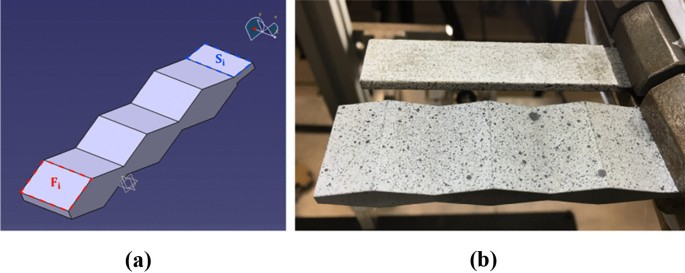Fig. 17

To perform the first calibration step, the real model of the manufactured calibration target was identified. First, a 3D surface measurement was performed using a Coordinate-Measuring Machine. For each main facet of the object (inclined planes, denoted as $$F_i$$ in Fig. 17(a)), the space coordinates of 50 points, uniformly distributed over the surface, were acquired. For each of the two small surfaces (horizontal planes $$S_i$$), 10 points were acquired.

A total of 620 points was used to build the model of the calibration target. Then, an algorithm based on least squares and least distances methods was implemented to find the best surface interpolating all the points of a facet . It is a two-step algorithm, where the first step was based on least squares fit between the measurements and the estimated plane, and the second step on the least distances method to update the plane normal direction. With such procedure, a more faithful parameterization of the surface is achieved in comparison to the nominal shape, and minimizes the distance of the measured points to the sought plane. Once the optimization of each face model was performed, all planes were combined to determine the corners of every facet. Then, the global model for the calibration target was constructed and can be expressed in different frameworks, either using an FE (T3) mesh or NURBS patches (Fig. 18).Fig. 18

### Appendix B: Calibration of the Two Cameras

The calibration procedure aims to minimize the cost function 

\begin{aligned} \phi ^2_c([{\varvec{P}}^1],[{\varvec{P}}^2])= & {} \sum _{j=1}^{n_s}~\sum _{{\varvec{X}}_j \in {\mathcal S_j}} \left( \frac{I_0^1({\varvec{x}}^1({\varvec{X}}_j,[{\varvec{P}}^1]))}{2(\sigma ^1)^2} \right. \nonumber \\&\left. - \frac{I_0^2({\varvec{x}}^2({\varvec{X}}_j,[{\varvec{P}}^2]))}{2(\sigma ^2)^2} \right) ^2 \end{aligned}
(28)

with respect to the components of the projection matrices $$[{\varvec{P}}^{1}]$$ and $$[{\varvec{P}}^{2}]$$, where $$\mathcal S_j$$ denotes the $$n_s=6$$ surfaces defining one side of the calibration target. The calibration procedure used herein was based an the FE formulation of global stereocorrelation . A fine mesh of the calibration target was considered. It was composed of 558 T3 elements 1.7 mm in size (Fig. 19). The total number of integration points was equal to 528 (i.e., $$N_{eq}\approx 23$$) so that the projected surface of one integration point had the size of roughly one pixel in the images (Table 3).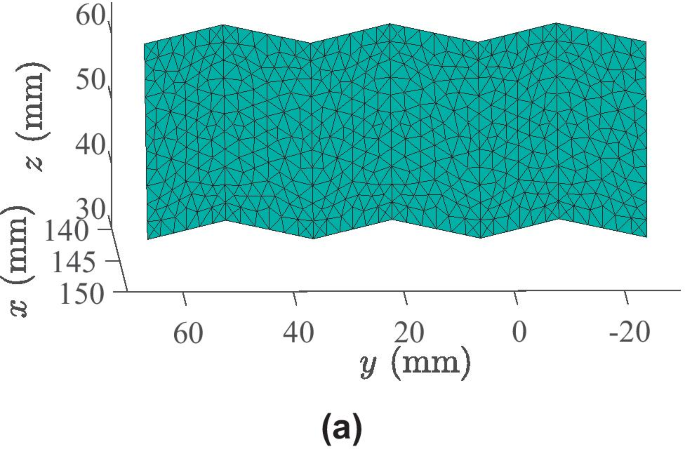Fig. 19

The minimization procedure was initialized with a direct estimation (via SVD [12, 48]) of the projection matrices, using the 3D positions of the 14 fiducials (i.e.,corners of the calibration target) and their associated projection in each image, see Fig. 20.Fig. 20

The associated gray level (GL) residuals using this initialization of the projection matrices are shown in Fig. 21(a) for each camera. The initialization led to a medium level of RMS residuals ($$\approx 21.4$$ gray levels, i.e.,8.4% of the dynamic range) and to errors on the description of the calibration target edges. This was expected since the initialization was obtained by using only 14 fiducials, which were manually selected on the images.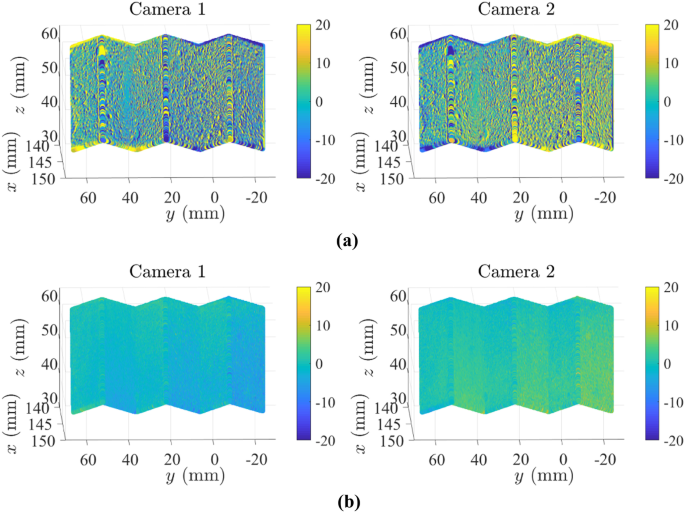Fig. 21

At the end of the optimization procedure, the calibration converged to a solution that considerably reduced the residuals (Fig. 21(b)) to an RMS level of 3.1 gray levels (i.e.,1.2% of the dynamic range). As opposed to Fig. 21(a), the edges were well-defined and the errors on the speckled parts were erased, hence leading to a good stereosystem calibration. Figure 22 illustrates how each node was correctly projected onto each image plane and matched the calibration target shapes on the images (especially for the edges, the corners and the inclined planes).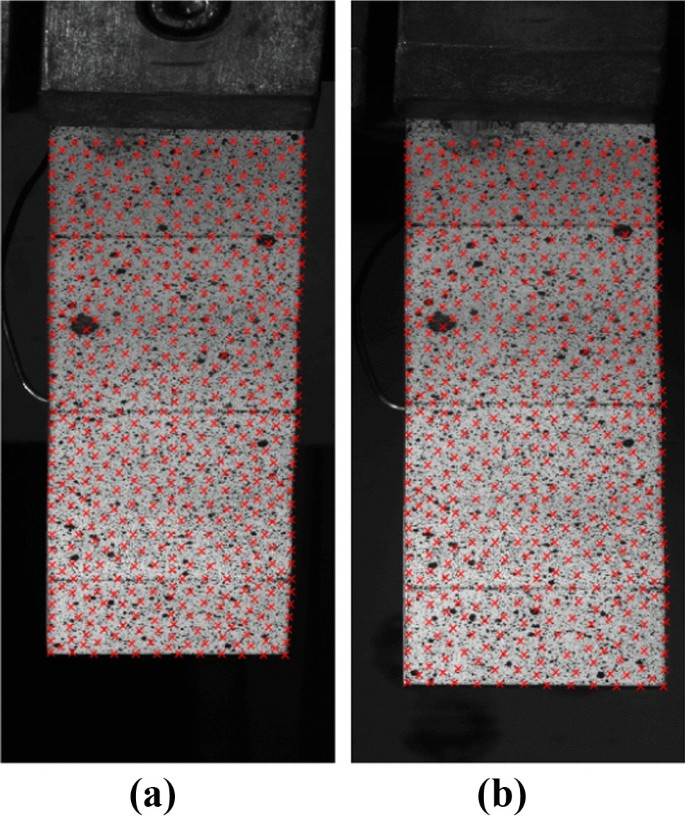Fig. 22

### Appendix C: Shape Correction for the CMC Sample

Displacements were then measured for the top surface of the CMC sample. The first step consisted in expressing its model in the calibration target frame. The correction procedure seeks to determine the (new) nodal positions gathered in the column vector $$\{{\varvec{N}}\}$$ of the surface mesh by globally minimizing the cost function .

\begin{aligned} \phi ^2_s(\{{\varvec{N}}\})= & {} \sum _{j=1}^{n_s}~\sum _{{\varvec{X}}_j \in {\mathcal S_j}} \left( \frac{I_0^1({\varvec{x}}^1({\varvec{X}}_j,\{{\varvec{N}}\}))}{2(\sigma ^1)^2} \right. \nonumber \\&- \left. \frac{I_0^2({\varvec{x}}^2({\varvec{X}}_j,\{{\varvec{N}}\}))}{2(\sigma ^2)^2} \right) ^2 \end{aligned}
(29)

Since the goal was to correct the global position of the CMC surface with respect to the calibration target, the FE mesh was very simple (i.e.,made of two T3 elements covering the CMC surface). Because the discretization was coarse, a large number of integration points (i.e.,8,256 corresponding to $$N_{eq}\approx 91$$) was selected. The nodal positions were initialized using in-situ distances between the sample and the calibration target. This initial model led to an RMS residual of 12.1 gray levels, corresponding to 4.7% of the dynamic range. The maps show that the residuals were higher on the right part (free edge) of the sample with a line of evaluation points with very high residuals (Fig. 23(a)). They indicated that the real model of the CMC sample was shorter and needed to be corrected.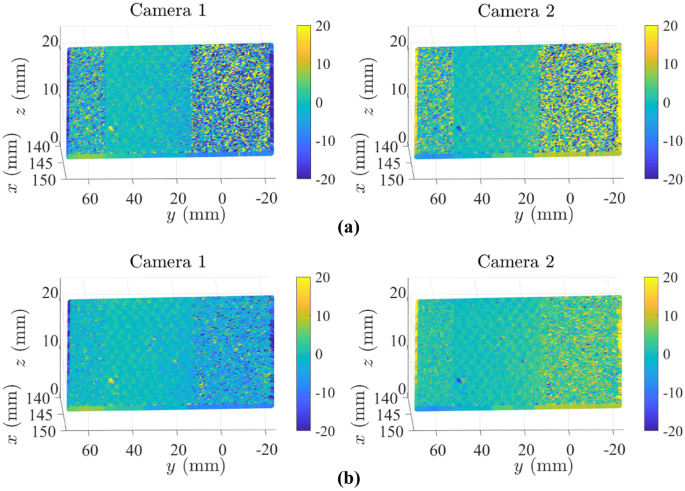Fig. 23

At convergence of the approach, the RMS residuals were reduced to 2.1% of the dynamic range (i.e.,5.3 gray levels) and the previous errors at the free edge were virtually erased (Fig. 23(b)). It was even possible to see the residuals associated with CMC 3D woven architecture of the central unspeckled part of the sample, which was a further proof of the good quality of the shape corrections.

The corrected position of the sample is shown in Fig. 24. The main contribution is on the out-of-plane component $$U_x$$ with a mean translation due to a wrong estimation of the sample thickness. There is also a gradient of $$\approx ~$$180 µm between the X-position of nodes in the clamp (corresponding to $$Y\approx 60$$ mm) and the nodes of the free edge, explained by the fact that the CMC sample was slightly inclined at the free edge. Regarding the components on the longitudinal Y and transverse Z-directions, the corrections mainly corresponded to an adjustment of the shape in terms of length and width.Fig. 24

Once the position of the sample was corrected, it was possible to measure its 3D surface displacements. A fine mesh was used with 498 T3 elements of mean size 1.5 mm (Table 3). To avoid convergence issues on displacement measurements, this mesh did not contain the unspeckled part and a line of nodes at the clamped edge, since they were hidden on one camera plane. Figure 25 shows the mesh with its projection onto both camera planes.Fig. 25

With this last step, the cameras were calibrated and the reference configuration of the CMC surface was expressed in the same frame. Displacement fields of the CMC surface and the calibration target can now be measured and are reported in a unique frame.

## Rights and permissions

Reprints and Permissions